Home

# ON PARTITIONS OF NONNEGATIVE INTEGERS AND REPRESENTATION FUNCTIONS

## Abstract

Let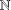$\mathbb{N}$ be the set of all nonnegative integers. For any set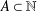$A\subset \mathbb{N}$ , let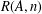$R(A,n)$ denote the number of representations of$n$ as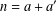$n=a+a^{\prime }$ with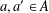$a,a^{\prime }\in A$ . There is no partition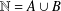$\mathbb{N}=A\cup B$ such that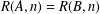$R(A,n)=R(B,n)$ for all sufficiently large integers$n$ . We prove that a partition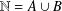$\mathbb{N}=A\cup B$ satisfies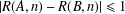$|R(A,n)-R(B,n)|\leq 1$ for all nonnegative integers$n$ if and only if, for each nonnegative integer$m$ , exactly one of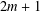$2m+1$ and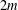$2m$ is in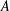$A$ .

## Footnotes

Hide All

The author is supported by the National Natural Science Foundation of China, Grant No. 11771211.

## References

Hide All
MathJax
MathJax is a JavaScript display engine for mathematics. For more information see http://www.mathjax.org.

# ON PARTITIONS OF NONNEGATIVE INTEGERS AND REPRESENTATION FUNCTIONS

## Metrics

### Full text viewsFull text views reflects the number of PDF downloads, PDFs sent to Google Drive, Dropbox and Kindle and HTML full text views.

Total number of HTML views: 0
Total number of PDF views: 0 *Loading metrics...

### Abstract viewsAbstract views reflect the number of visits to the article landing page.

Total abstract views: 0 *Loading metrics...

* Views captured on Cambridge Core between <date>. This data will be updated every 24 hours.

Usage data cannot currently be displayed